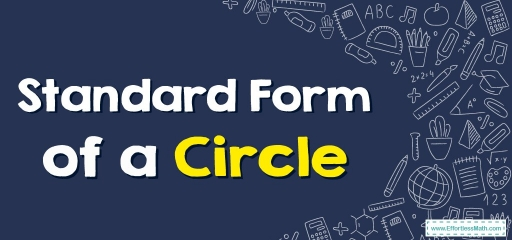# Standard Form of a Circle

The equation of a circle is written using the radius and center of the circle.The equation of the circle is shown with the center and radius of the circle. With this information, we can sketch the graph of the circle.

## Step by Step Guide to Write the Standard Form of a Circle

• The Standard form of a circle is $$(x- h)^2+( y-k)^2= r^2$$, where $$r$$ is the radius and $$(h, k)$$ is the center. By knowing the center and radius of the circle we can write the standard form of a circle.

### Standard form of a Circle – Example 1:

Write the standard form equation of circle with center: $$(0, 5)$$, radius: $$3$$

Solution:

The Standard form of a circle is $$(x- h)^2+( y-k)^2= r^2$$, where $$r$$ is the radius and $$(h, k)$$ is the center.

In this case, the center is $$(0, 5)$$ and the radius is $$3$$: $$(h, k)=(0, 5), r=3$$

Then: $$(x- 0)^2+( y-5)^2= 3^2 → x^2+( y-5)^2= 9$$

### Standard form of a Circle– Example 2:

Write the standard form equation of the circle $$x^2+y^2-6x+2y= 6$$.

Solution:

The Standard form of a circle is $$(x- h)^2+( y-k)^2= r^2$$, where $$r$$ is the radius and $$(h, k)$$ is the center.

Group $$x$$-variables and $$y$$-variables together: $$(x^2-6x)+( y^2+2y)= 6$$

Convert $$x$$ to square form: $$(x^2-6x+9)+( y^2+2y)= 6+9 → (x-3)^2+( y^2+2y)=6+9$$

Convert $$y$$ to square form: $$(x-3)^2+( y^2+2y+1)= 6+9+1 → (x-3)^2+(y+1)^2=6+9+1$$

Then: $$(x-3)^2+(y+1)^2=4^2$$

## Exercises for Writing Standard form of a Circle

### Write the standard form equation of each circle with the given information.

• $$\color{blue}{Center: (0, 4)}, \color{blue}{Radius: 2}$$
• $$\color{blue}{Center: (-1, 2)}$$, $$\color{blue}{Radius: 5}$$
• $$\color{blue}{x^2+y^2-6x+8y=0}$$
• $$\color{blue}{x^2+y^2-2x+8y=0}$$
• $$\color{blue}{x^2+(y-4)^2=2^2}$$
• $$\color{blue}{(x+1)^2+(y-2)^2=5^2}$$
• $$\color{blue}{(x-5)^2+y^2=4^2}$$
• $$\color{blue}{(x-1)^2+(y+4)^2=5^2}$$

### What people say about "Standard Form of a Circle - Effortless Math: We Help Students Learn to LOVE Mathematics"?

No one replied yet.

X
30% OFF

Limited time only!

Save Over 30%

SAVE $5 It was$16.99 now it is \$11.99